# ContextFree Languages 1 Regular Languages 2 ContextFree Languages

• Slides: 74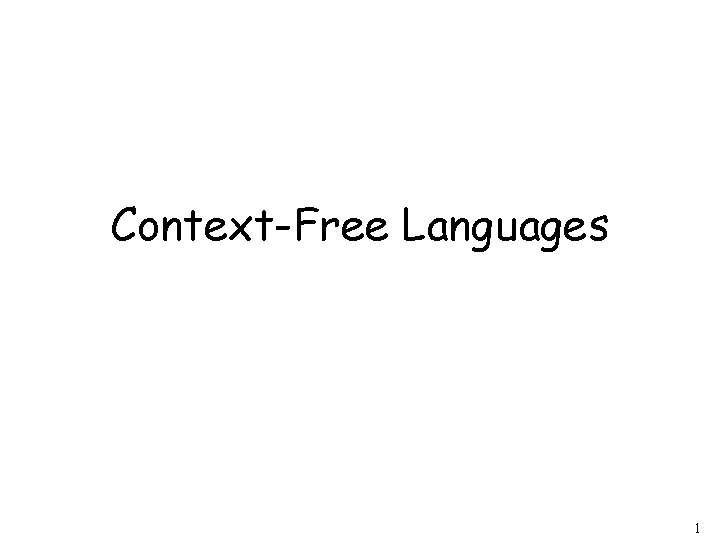Context-Free Languages 1Regular Languages 2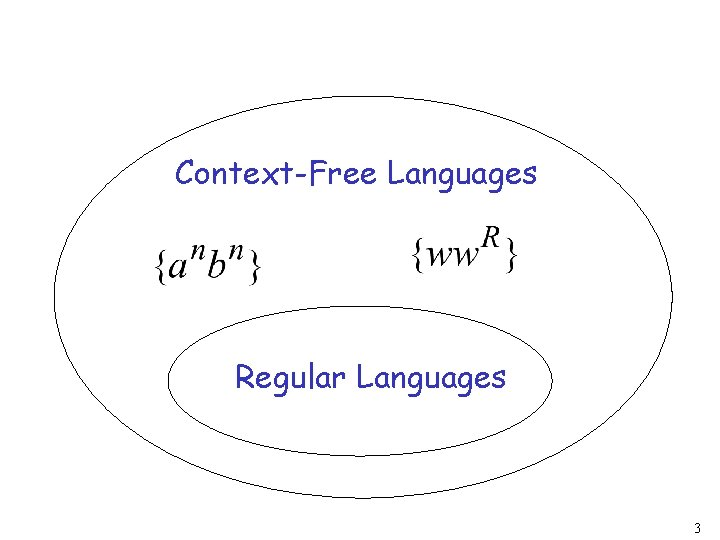Context-Free Languages Regular Languages 3Context-Free Languages Context-Free Grammars Pushdown Automata stack automaton 4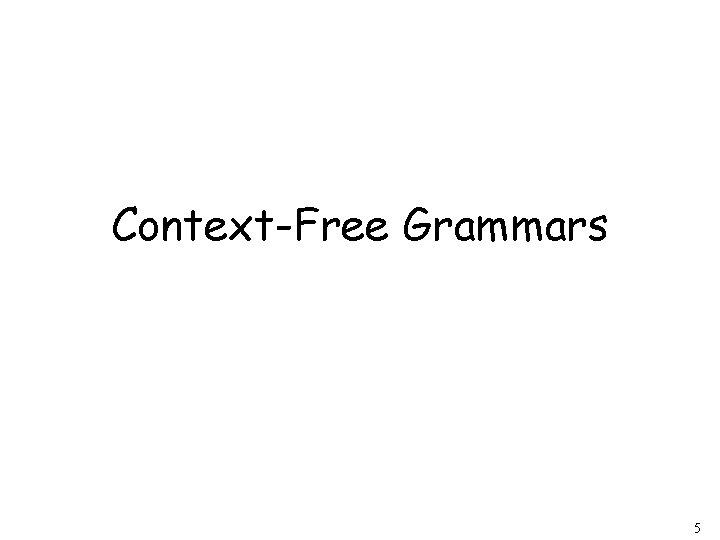Context-Free Grammars 5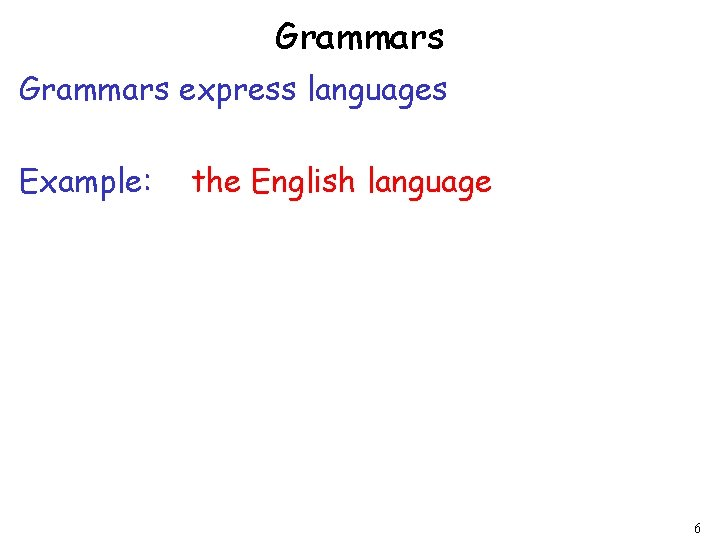Grammars express languages Example: the English language 6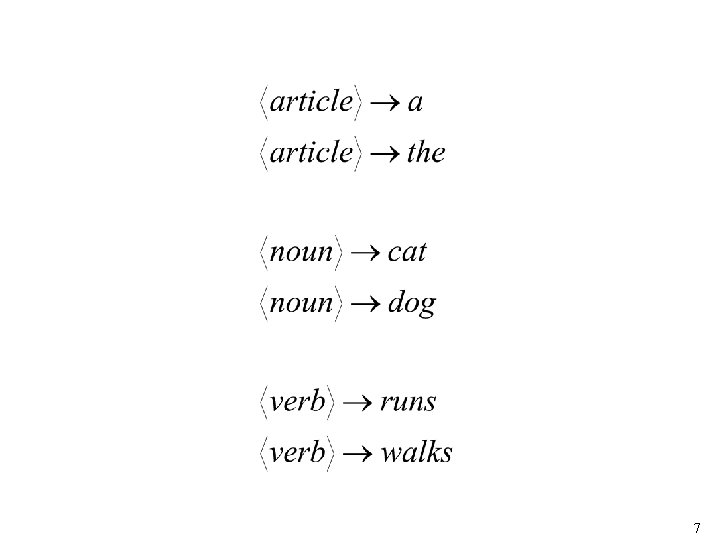7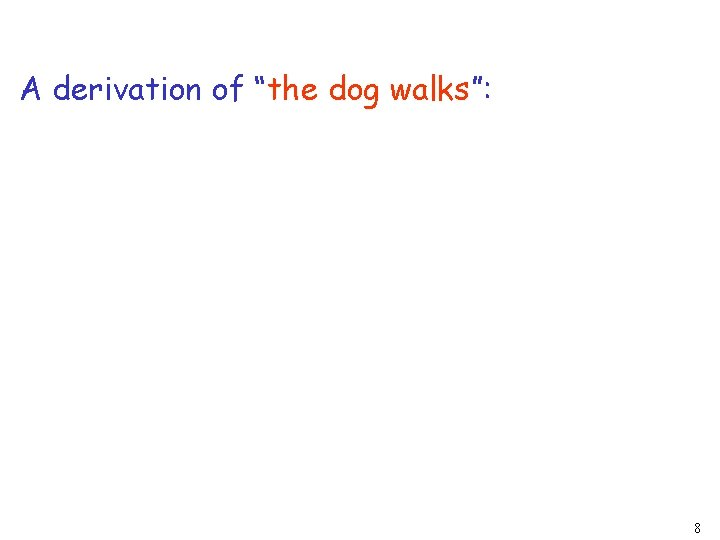A derivation of “the dog walks”: 8A derivation of “a cat runs”: 9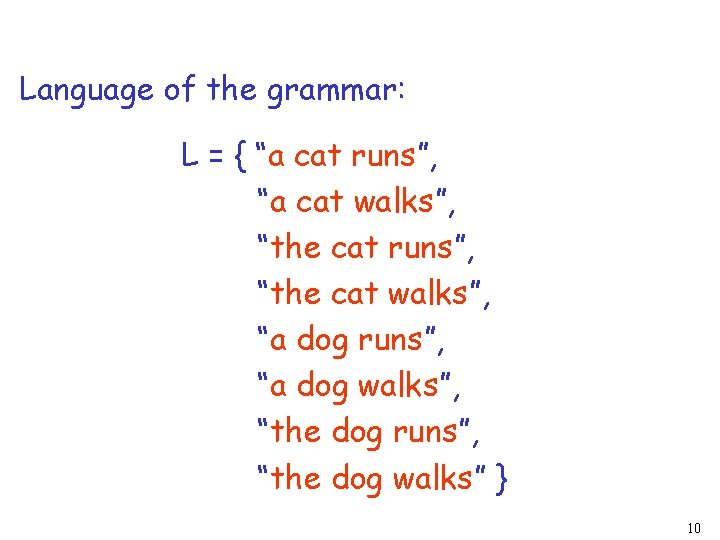Language of the grammar: L = { “a cat runs”, “a cat walks”, “the cat runs”, “the cat walks”, “a dog runs”, “a dog walks”, “the dog runs”, “the dog walks” } 10Notation Production Rules Variable Terminal 11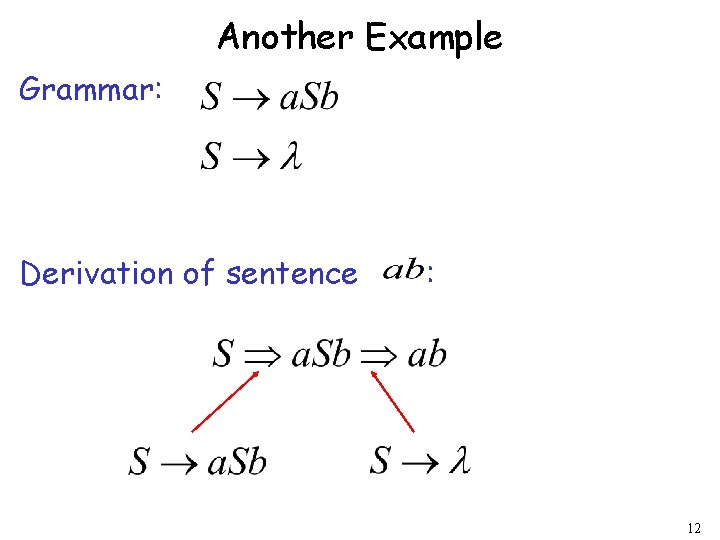Another Example Grammar: Derivation of sentence : 12Grammar: Derivation of sentence : 13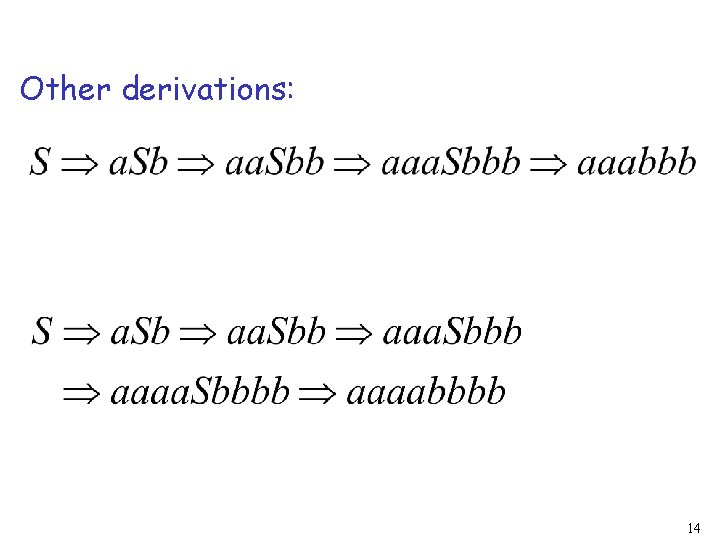Other derivations: 14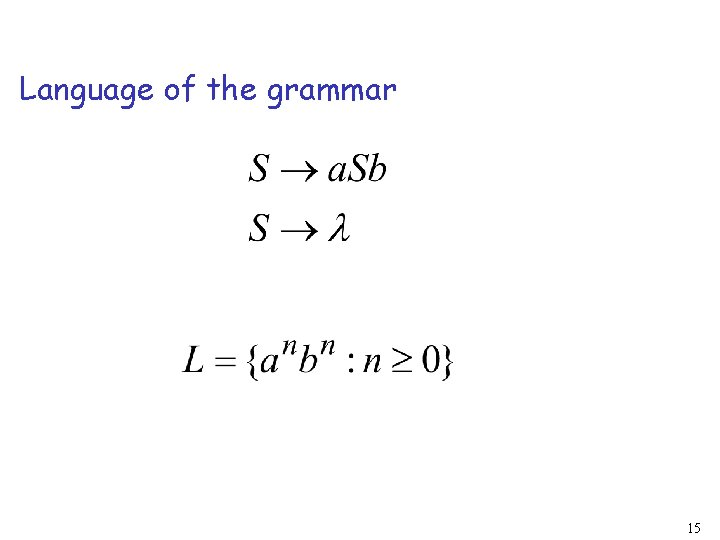Language of the grammar 15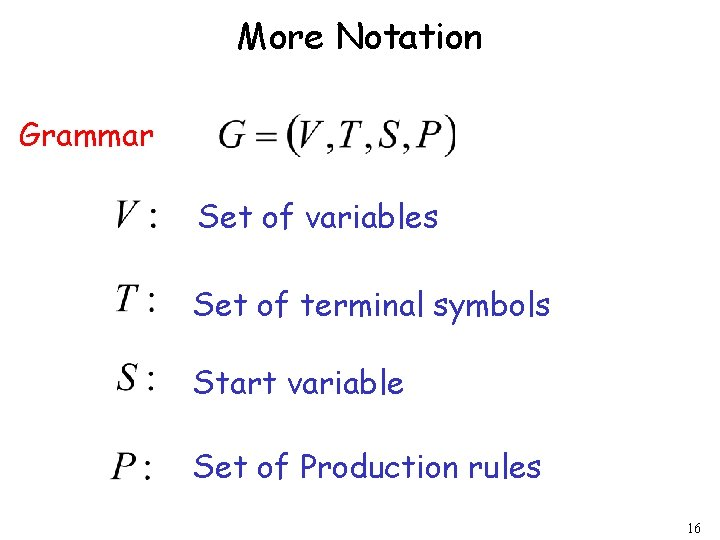More Notation Grammar Set of variables Set of terminal symbols Start variable Set of Production rules 16Example Grammar : 17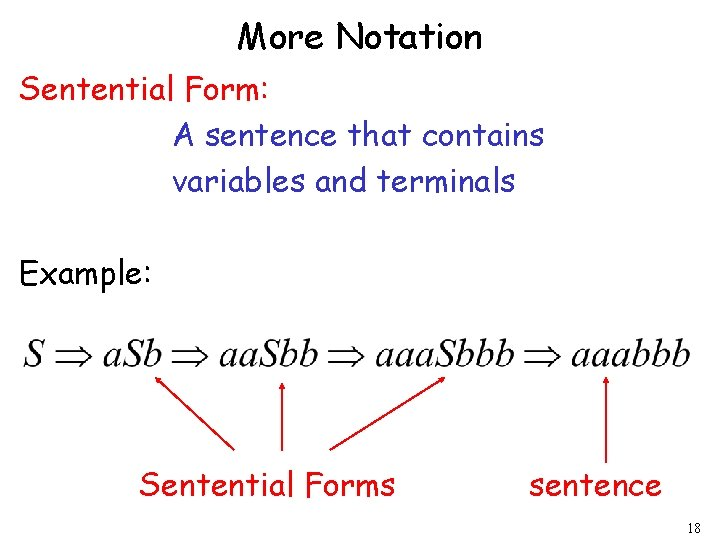More Notation Sentential Form: A sentence that contains variables and terminals Example: Sentential Forms sentence 18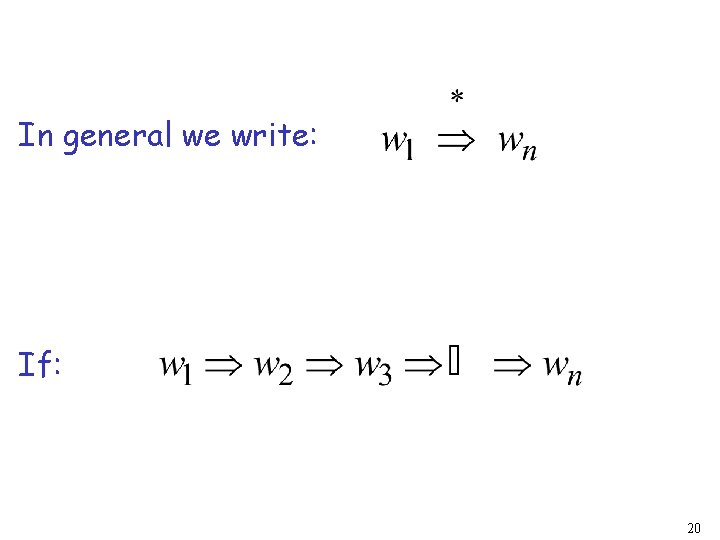In general we write: If: 20By default: 21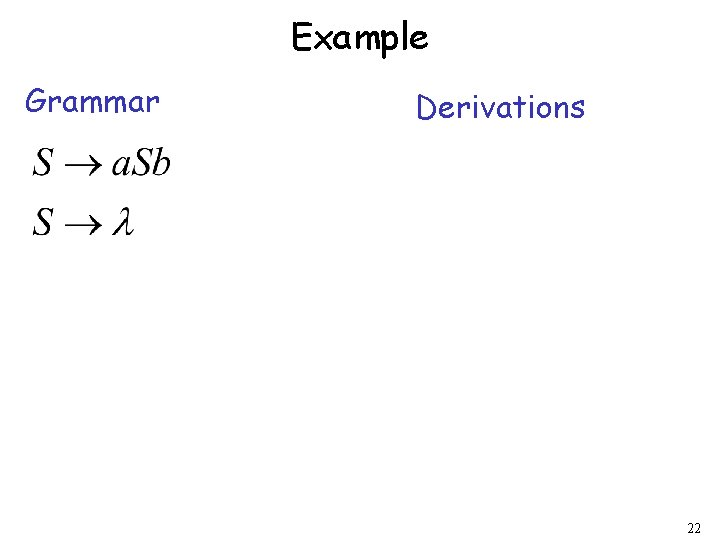Example Grammar Derivations 22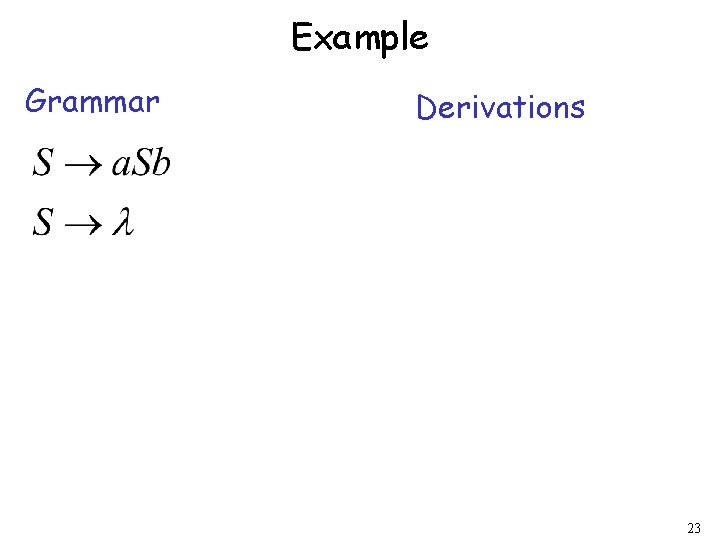Example Grammar Derivations 23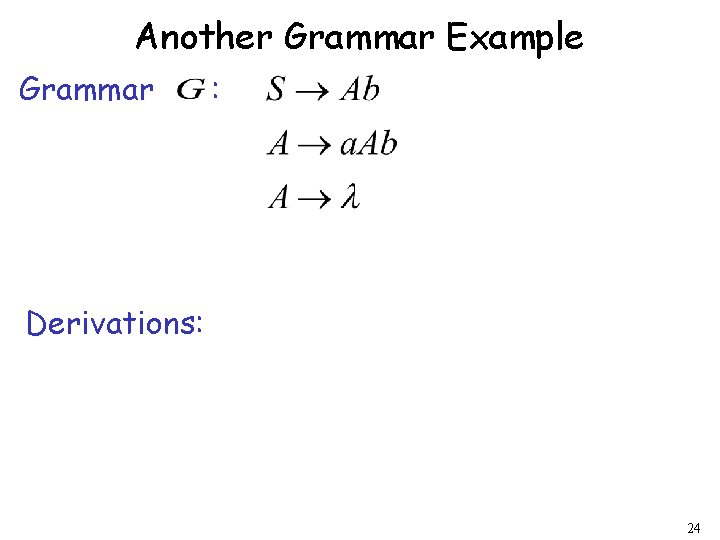Another Grammar Example Grammar : Derivations: 24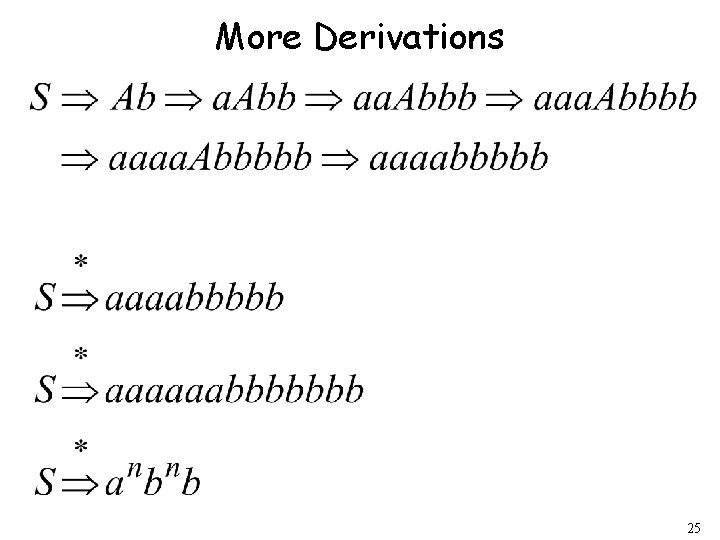More Derivations 25Language of a Grammar For a grammar with start variable : String of terminals 26Example For grammar : Since: 27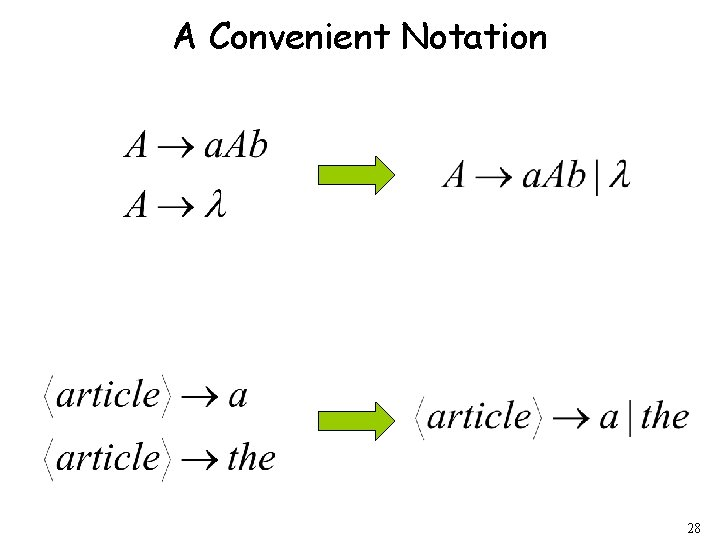A Convenient Notation 28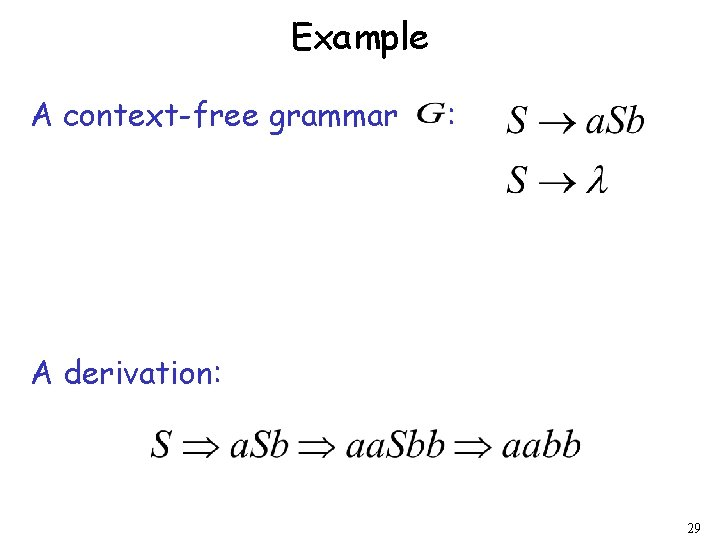Example A context-free grammar : A derivation: 29A context-free grammar : Another derivation: 30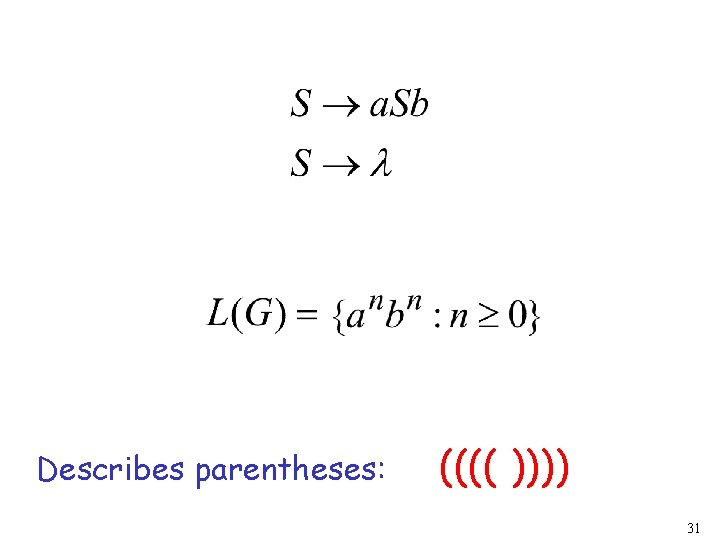Describes parentheses: (((( )))) 31Example A context-free grammar : A derivation: 32A context-free grammar : Another derivation: 3334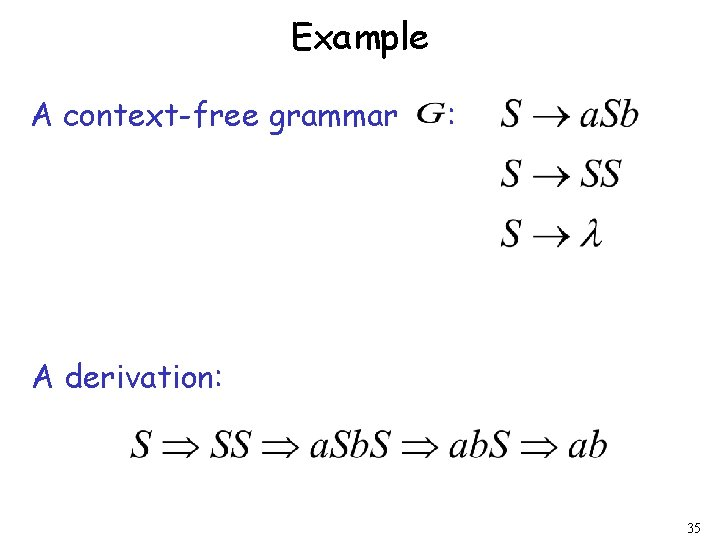Example A context-free grammar : A derivation: 35A context-free grammar : A derivation: 36Describes matched parentheses: () ((( ))) (( )) 37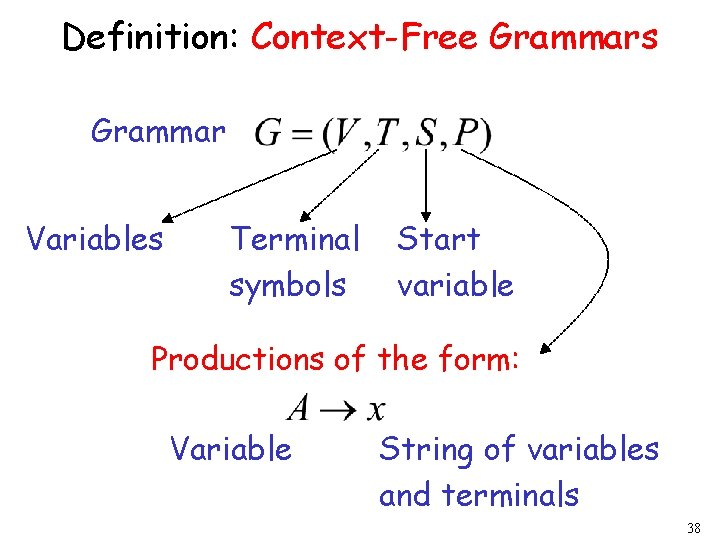Definition: Context-Free Grammars Grammar Variables Terminal symbols Start variable Productions of the form: Variable String of variables and terminals 38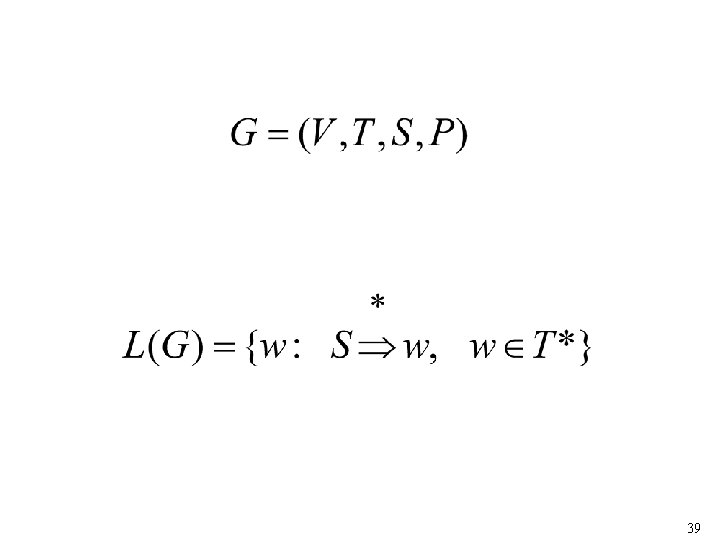39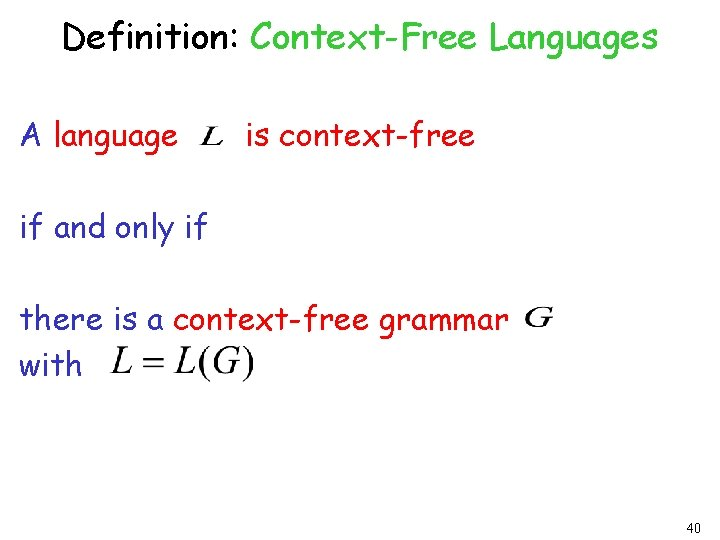Definition: Context-Free Languages A language is context-free if and only if there is a context-free grammar with 40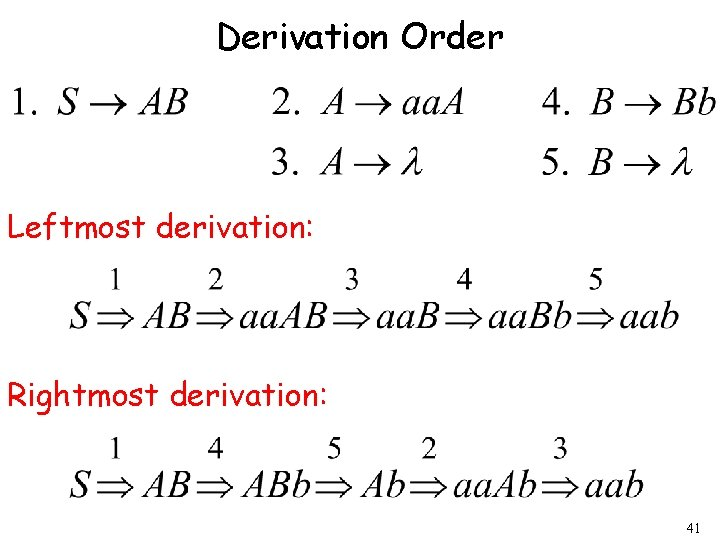Derivation Order Leftmost derivation: Rightmost derivation: 41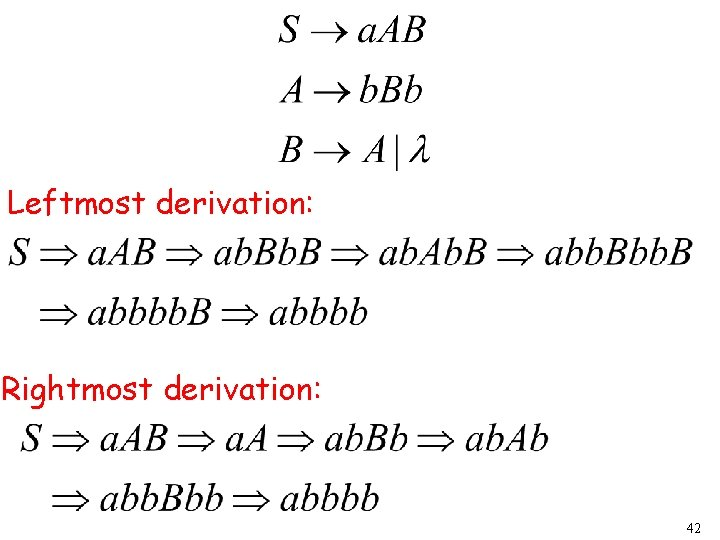Leftmost derivation: Rightmost derivation: 42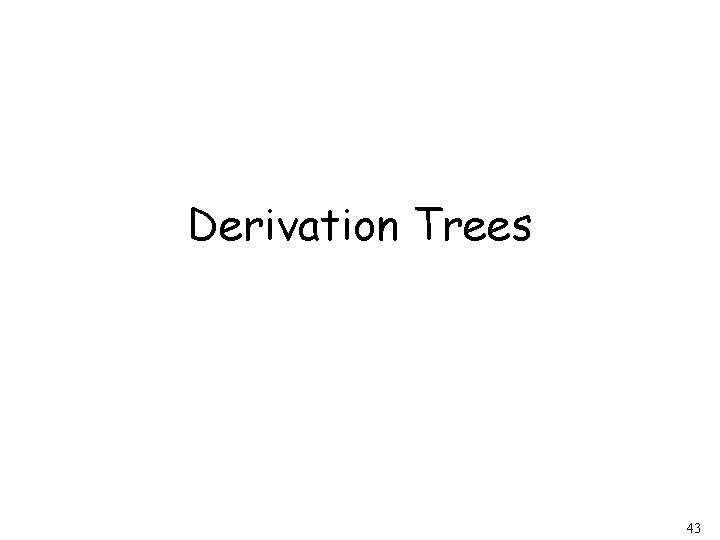Derivation Trees 4344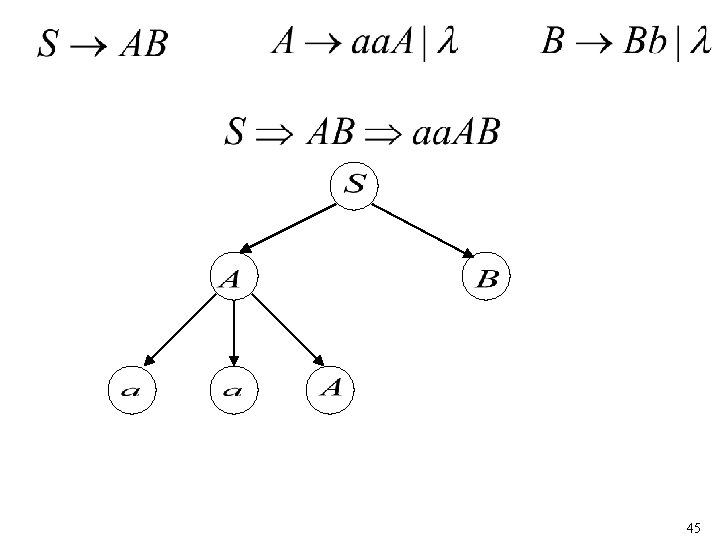4546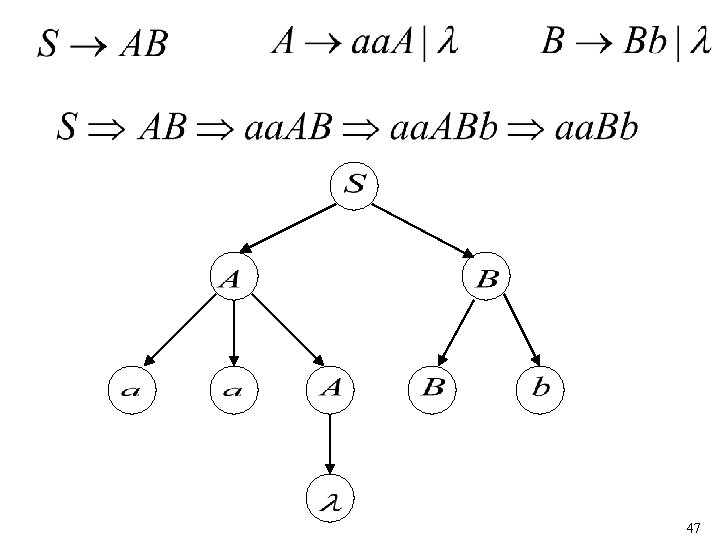47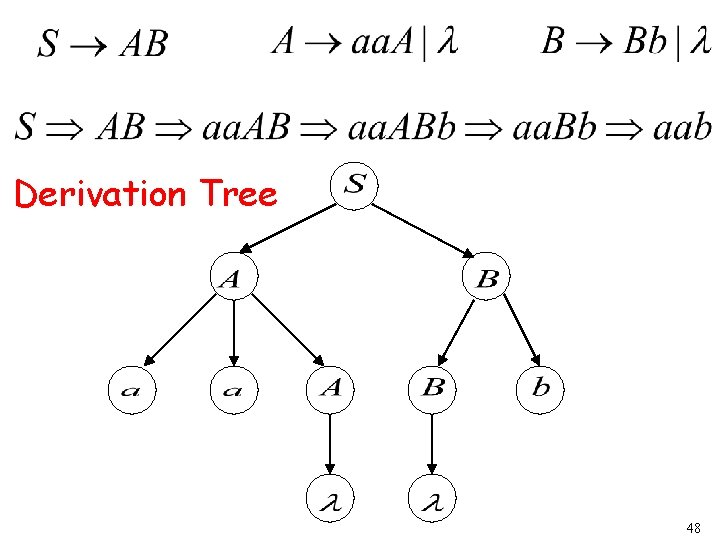Derivation Tree 48Derivation Tree yield 49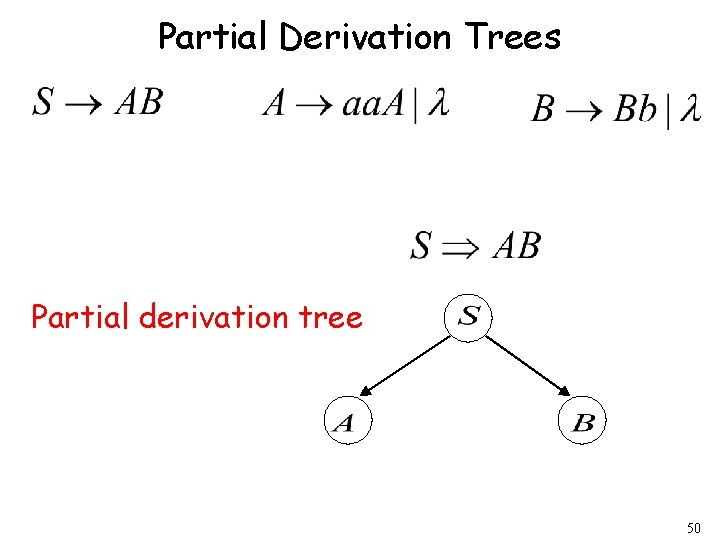Partial Derivation Trees Partial derivation tree 50Partial derivation tree 51sentential form Partial derivation tree yield 52Sometimes, derivation order doesn’t matter Leftmost: Rightmost: Same derivation tree 53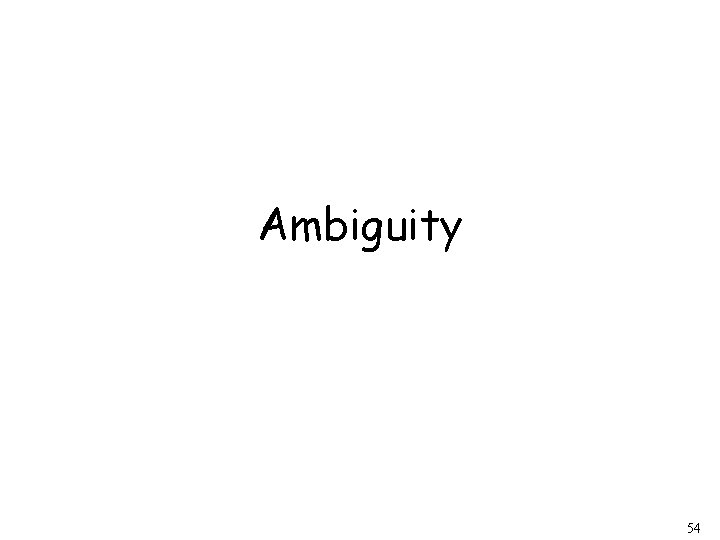Ambiguity 54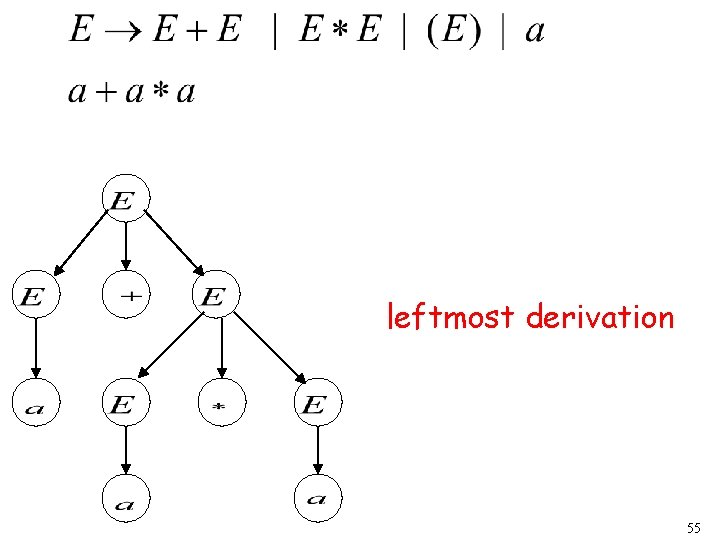leftmost derivation 55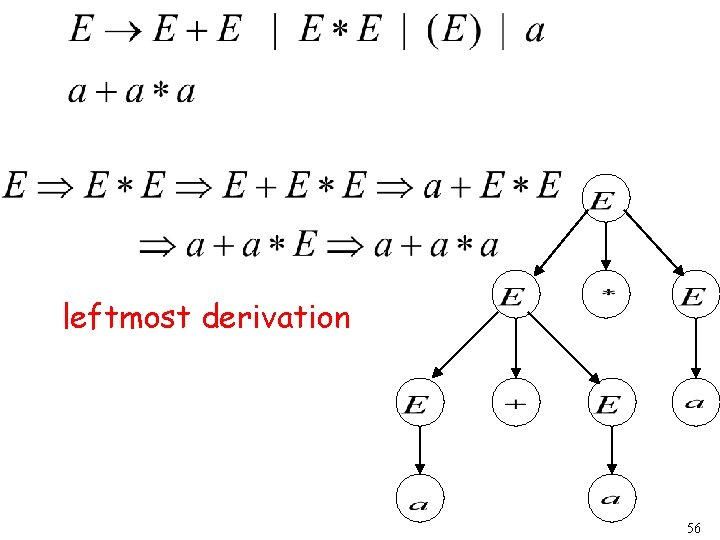leftmost derivation 56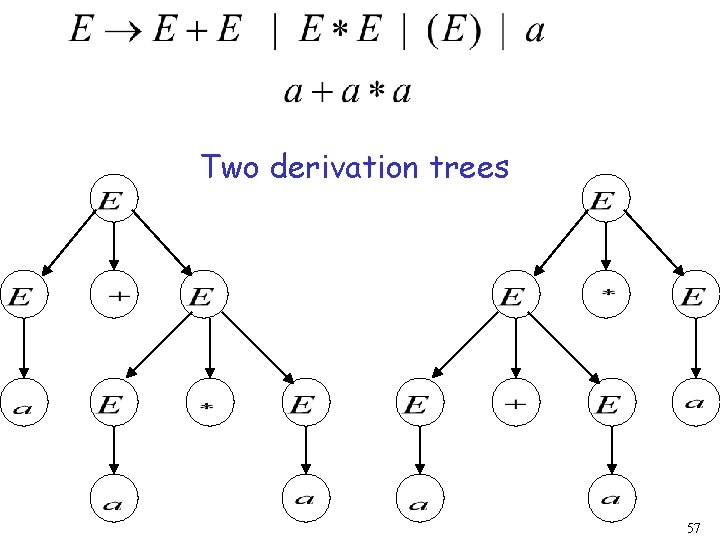Two derivation trees 57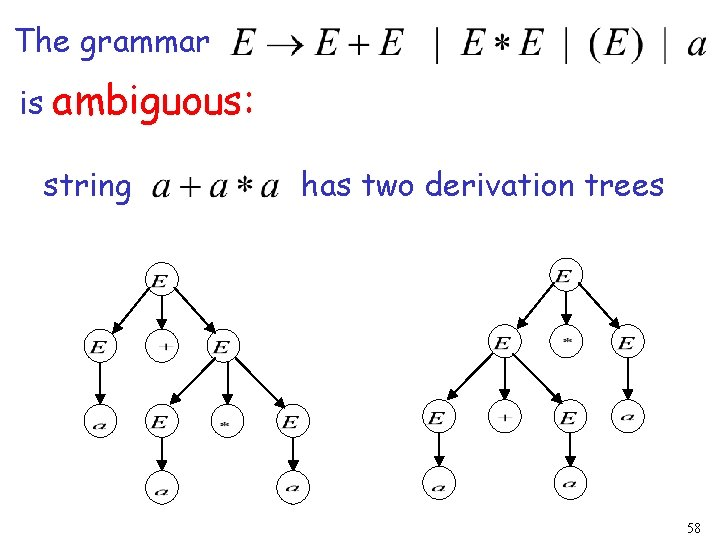The grammar is ambiguous: string has two derivation trees 58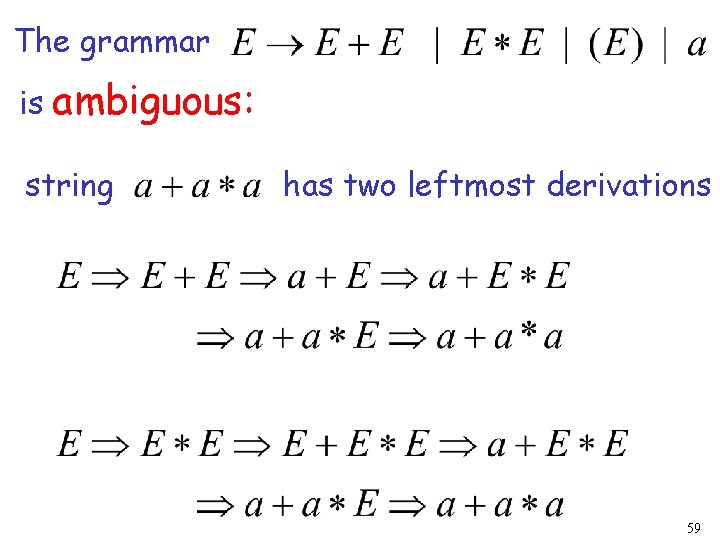The grammar is ambiguous: string has two leftmost derivations 59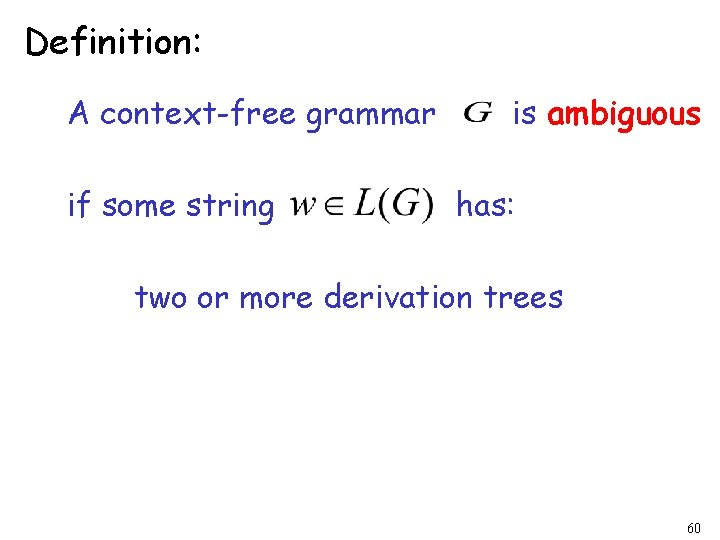Definition: A context-free grammar if some string is ambiguous has: two or more derivation trees 60In other words: A context-free grammar if some string is ambiguous has: two or more leftmost derivations (or rightmost) 61Why do we care about ambiguity? take 62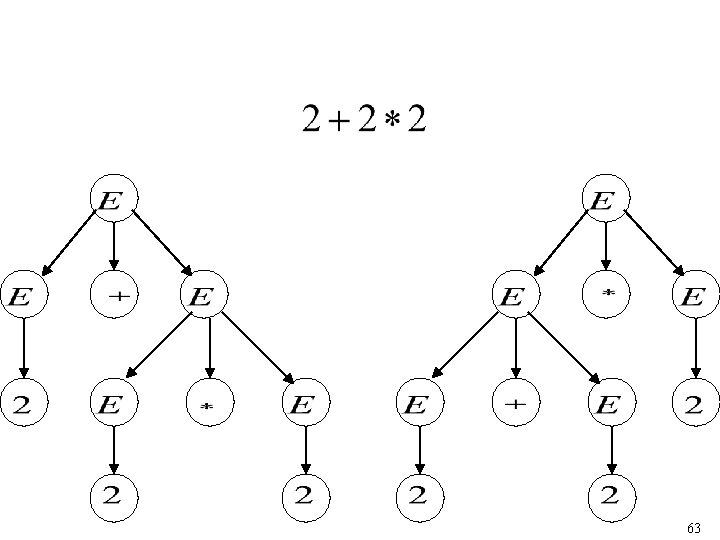6364Correct result: 65• Ambiguity is bad for programming languages • We want to remove ambiguity 66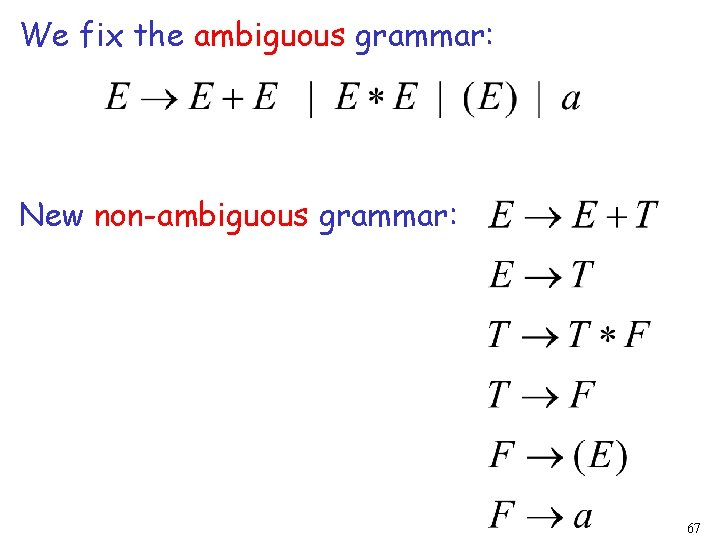We fix the ambiguous grammar: New non-ambiguous grammar: 6768Unique derivation tree 69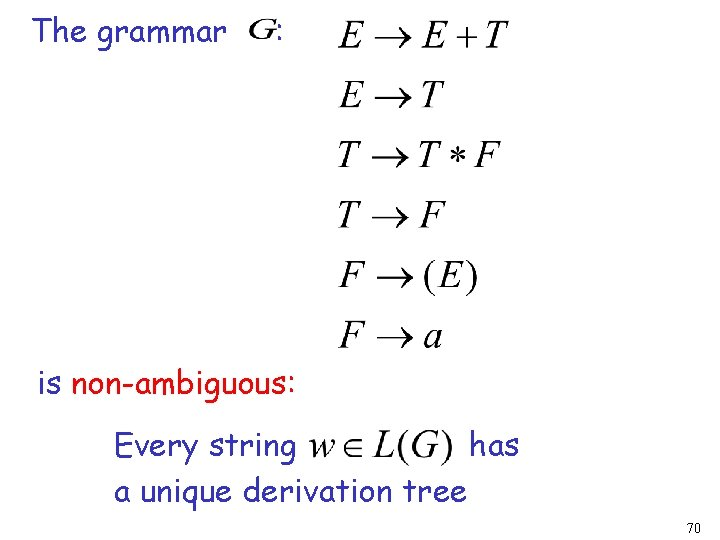The grammar : is non-ambiguous: Every string has a unique derivation tree 70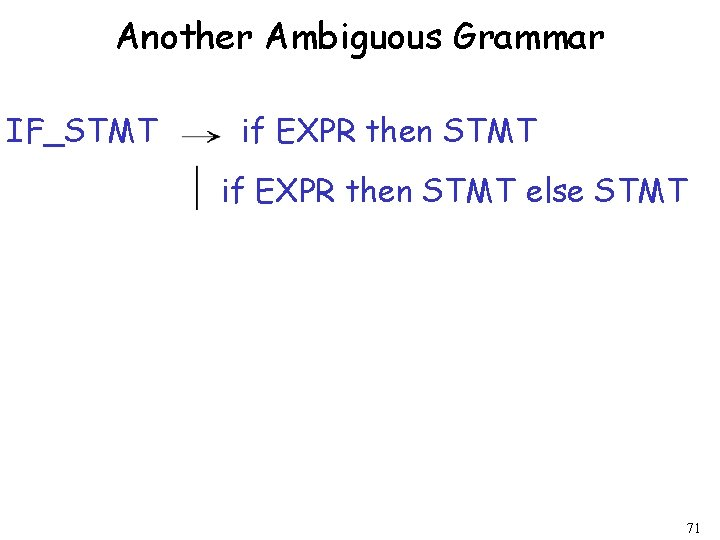Another Ambiguous Grammar IF_STMT if EXPR then STMT else STMT 71If expr 1 then if expr 2 then stmt 1 else stmt 2 IF_STMT if expr 1 if then expr 2 STMT then stmt 1 else STMT else stmt 2 IF_STMT if expr 1 if then expr 2 then stmt 1 72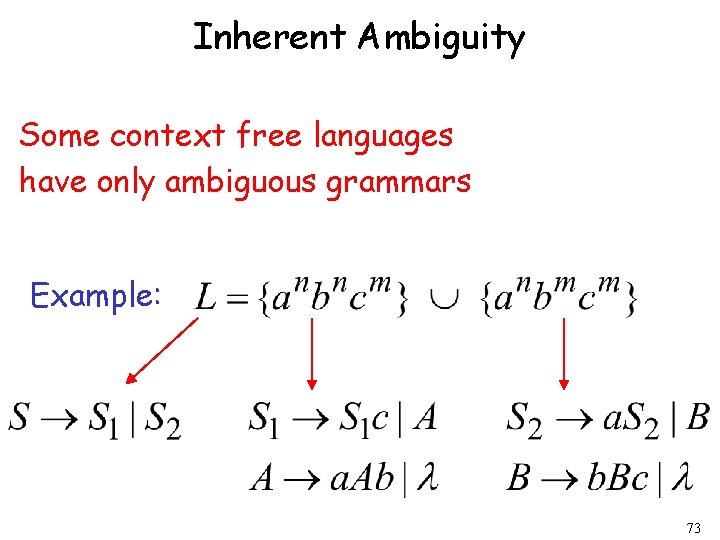Inherent Ambiguity Some context free languages have only ambiguous grammars Example: 73The string has two derivation trees 74